# SAS/ETS Web Examples

## Calculating Economic Indices

Contents | SAS Program

# Overview

This example illustrates the calculation of some widely known economic indices such as Laspeyre, Paasche, Bowley, Fisher, and more by defining them with PROC FCMP and then accessing the compiled functions by using the SAS global option CMPLIB in a DATA step. An economic index is a statistic about the economy that is used to study relative movements in prices or quantities over a period of time. They assist in decision-making to create a stable economy by predicting future performance.

Some of the popular ways of computing economic indices are given by Laspeyre’s index, Paasche’s index, Bowley’s index, and Fisher’s index formulas. Each of the index formulas can be used to compute both a price index and a quantity index. A price index measures the change of price over time for a fixed basket of products and services. A quantity index calculates the change in consumption over time for a basket of goods with a fixed value at a certain time. Some examples of price-related economic indices are the consumer price index (CPI), import and export price indices, producer price indices, and the employment cost index. The growth rate of gross domestic product (GDP) is an example of quantity related change. The following indices are calculated in this example:

• Laspeyre’s
• Paasche’s
• Bowley’s
• Fisher’s
• Marshall Edgeworth’s
• Mitchell’s
• Walsh’s
• geometric mean
• harmonic mean

# Details

An ideal index might be expected to have a fixed weight in the numerator and denominator. But with a price change, quantities purchased are rarely identical over two given periods. Although Laspeyre’s and Paasche’s index formulas are two widely used methods to calculate indices, these indices do not account for the fact that the consumers typically react to price changes by changing the quantities they purchase. With an increase in price, the consumer would reduce the quantity; hence the weights added to the Paasche’s index would be smaller than that of Laspeyre’s index. As a result, Paasche’s index systematically understates inflation while Laspeyre’s index overstates it.

To compensate for this discrepancy, different formulas were introduced. Bowley’s and Fisher’s formulas use the arithmetic mean and the geometric mean (of the Laspeyre’s and Paasche’s index values), respectively. Marshall-Edgeworth’s formula uses an average of the base price and the current price as weights. The Fisher’s index is more appropriate when dealing with percentage changes. As their names suggest, the harmonic mean index computes the harmonic average and the geometric mean index computes the geometric mean.

Except for Laspeyre’s, the harmonic mean, and the geometric mean, all the other indices require revised knowledge of the current expenditure pattern. This is a disadvantage because collecting current information and updating weights require more time and effort. All the indices put together serve as an overall measure of the relative movements.

In the following formulas, let perioddenote the reference point in an earlier period, also sometimes known as the base period. Let perioddenote the current time of interest with which the base period is compared. Let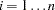denote the items in the basket over which the summation is carried out. Let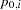denote the price of theth item at base period anddenote the price of theth item at the current period of interest. Let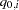denote the quantity of theth item at the base period and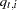denote the quantity of theth item at the current period of interest. Some of the fixed-weight price indices illustrated in this example and defined by using PROC FCMP are enumerated below. The function names defined in PROC FCMP are shown in parentheses. For each index type, the quantity index formula is defined, analogous to its corresponding price index formula.

• Laspeyre’s

• price index (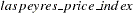)• quantity index ()• Paasche’s

• price index ()• quantity index ()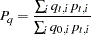• Bowley’s

• price index (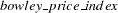)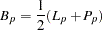• quantity index (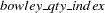)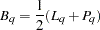• Fisher’s

• price index ()• quantity index ()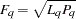• geometric mean (GM)

• price index (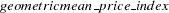)• quantity index (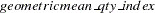)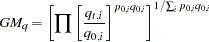• harmonic mean (HM)

• price index (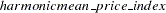)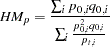• quantity index (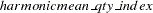)• Marshall-Edgeworth’s

• price index (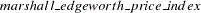)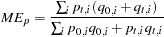• quantity index (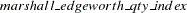)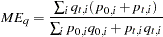• Walsh’s

• price index ()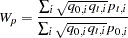• quantity index (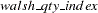)• Mitchell’s

• price index (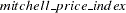)• Mitchell’s quantity index ()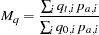Thein the Mitchell’s index denotes the relative importance of the items. Mitchell has advocated using the average of quantities bought and sold over a period of time to be used as weights (Kenney and Keeping 1962). In this example, the Mitchell’s price index function is calculated with the weight "wt" (defined asin the preceding formula) as the average ofand. You can supply your own weights depending on how you rank the items in their relative order of importance. Instead of using the preceding Mitchell’s formula, some users might have a better idea of the "wt" given by value,of their products. In that case, Mitchell’s price index formula is given by:Similarly, Mitchell’s quantity index formula is given by: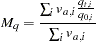where in this case,is defined as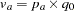.

Note that the price indices defined by using PROC FCMP can be used to compute the quantity indices as well, by interchanging the price and quantity values. To avoid confusion, the quantity index formulas are defined separately. For example, the Laspeyre’s price index needs input arguments of. You can obtain Laspeyre’s quantity index by changing the input arguments to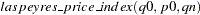, replacing the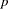’s with’s and vice versa. This yields the same result as using the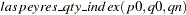.

# Analysis

You can define the index functions as subroutines by using the FCMP procedure. In this example, the functions are stored in "sasuser.ecoidx.economic_indicators" by using the OUTLIB option in the PROC FCMP statement. Thecreated by PROC FCMP is shown as follows:

```
%let OUTLIB = sasuser.ecoidx.economic_indicators;

/*-------- create functions with PROC FCMP --------*/

proc fcmp outlib= &OUTLIB;
function laspeyres_price_index( p0[*], q0[*], pn[*] ) label= "Laspeyres Price Index";
/*---------------------------------------------------------------------
* ENTRY:     laspeyres_price_index
*
* PURPOSE:   Computes Laspeyres Price index.
*
* USAGE:     idxl_p = laspeyres_price_index( p0, q0, pn );
*            p0 denotes price vector for items at time 0/base;
*            q0 denotes quantity vector for items at time 0/base;
*            pn denotes price vector for items at time n;
*            In the following example for 2 items,
*            the Laspeyres Price index has been calculated.
*
* NOTE:      Missing values as arguments to the function return missing value.
*
* EXAMPLES:  idxl_p = laspeyres_price_index( p0, q0, pn );
*
*
*--------------------------------------------------------------------*/
if  dim(p0) ~= dim(q0) | dim(p0) ~= dim(pn) then do;
Put "ERROR: Arguments to laspeyres_price_index do not have the same dimensions";
return( . );
end;
num=0;den=0;
do i=1 to dim(p0);
num=num + (pn[i]*q0[i]);
den=den + (p0[i]*q0[i]);
end;
idxl_p=num/den;
return( idxl_p );
endsub;
```

The variable IDXL_P holds the computed Laspeyre’s price index. The RETURN statement in a subroutine enables the computed result to be returned when the specified function is invoked. Note that the IDXL_P result is used later in a DATA step given below. You need the base price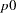, base quantity, and current priceto calculate the Laspeyre’s price index. The input arguments for thefunction are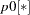,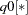, and. The
indenotes an array that contains the base prices for all the items in the basket. The other subroutines that define the other index functions have been created similarly. The complete statements can be found in the accompanying SAS source file(sas.html). The data set contains wine and cheese products over two time periods T1 and T2. The observations are as follows:

 Product T1 T2 types q p q p Cheese 100 15 108 18 Wine 25 22 38 16

The library catalog 'sasuser.ecoidx' in the SAS global option CMPLIB= specifies where to look for the previously compiled functions created in PROC FCMP. For more information about the FCMP procedure, see the Base SAS Procedures Guide, Version 9. The following DATA step reads the data and calculates the various price indices.

```   /*-------- test functions with datastep --------*/
/***calculate price index***/
options  CMPLIB= (sasuser.ecoidx);
data indices(drop=i);
array p0 _temporary_ (15 22);
array q0 _temporary_ (100 25);
array pn _temporary_ (18 16);
array qn _temporary_ (108 38);
array wt_q _temporary_;
array wt_p _temporary_;
do i =1 to dim(p0);
wt_q[i] = .5 * (q0[i]+ qn[i]);
wt_p[i] = .5 * (p0[i]+ pn[i]);
end;
idxl_p = laspeyres_price_index( p0, q0, pn );
idxp_p = paasche_price_index( p0, pn, qn );
idxb_p = bowley_price_index( p0, q0, pn, qn );
idxf_p = fisher_price_index( p0, q0, pn, qn );
idxgm_p = geometricmean_price_index( p0, q0, pn );
idxhm_p = harmonicmean_price_index( p0, q0, pn );
idxme_p = marshall_edgeworth_price_index( p0, q0, pn, qn );
idxw_p = walsh_price_index( p0, q0, pn, qn );
idxm_p = mitchell_price_index( p0, wt_q, pn, 1 ); /*<----user supplied _type_ */
idxl_q = laspeyres_qty_index( p0, q0, qn );
idxp_q = paasche_qty_index( q0, pn, qn );
idxb_q = bowley_qty_index( p0, q0, pn, qn );
idxf_q = fisher_qty_index( p0, q0, pn, qn );
idxgm_q = geometricmean_qty_index( p0, q0, qn );
idxhm_q = harmonicmean_qty_index( p0, q0, qn );
idxme_q = marshall_edgeworth_qty_index( p0, q0, pn, qn );
idxw_q = walsh_qty_index( p0, q0, pn, qn );
idxm_q = mitchell_qty_index( q0, wt_p, qn, 1 ); /*<----user supplied _type_ */
run;

```

Figure 3.1 shows the price indices computed with the Laspeyre’s, Paasche’s, Bowley’s, Fisher’s, geometric-mean, harmonic-mean, Marshall-Edgeworth’s, Walsh’s, and Mitchell’s index formulas.

Figure 3.1 Price Indices

Obs idxl_p idxp_p idxb_p idxf_p idxgm_p idxhm_p idxme_p idxw_p idxm_p
1 1.07317 1.03909 1.05613 1.05599 1.04914 1.02181 1.03259 1.05670 1.05459

Note that the relative importance of the items denoted by "wt" (orin the Mitchell’s price index formula) is calculated as an average of the base and the current quantities while computing price indices. You can supply your own weights "wt" based on your judgment of the relative importance of the items.

As pointed out earlier, if you are required to calculate the Laspeyre’s quantity index, you could enterwith’s replaced by’s and vice versa or. Note that during the quantity index calculation, the "wt" in Mitchell’s formula is calculated as an average of the base and the current prices. Again you might use theweighted Mitchell’s formula. In that case, you would enter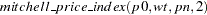instead of "1" for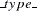. For the same dataset, the quantity indices are computed.

Figure 3.2 shows the various quantity indices computed for the same data with the Laspeyre’s, Paasche’s, Bowley’s, Fisher’s, geometric-mean, harmonic-mean, Marshall-Edgeworth’s, Walsh’s, and Mitchell’s index formulas.

Figure 3.2 Quantity Indices

Obs idxl_q idxp_q idxb_q idxf_q idxgm_q idxhm_q idxme_q idxw_q idxm_q
1 1.19805 1.16 1.17902 1.17887 1.18371 1.17094 1.08822 1.17771 1.17835

When a price increases, the price index increases; when the price falls, the index falls as well. The same is true for the quantity index. For the small data set presented here, you can guess what the results would be. Over the two time periods, there is an increase in the price of cheese and a decrease in the price of wine. However, the quantities purchased for both cheese and wine show an increase over the two periods. If you compare the two results above, the quantity index values are slightly larger than price index values; this is expected if you compare the relative changes by observation.

# References

SAS Institute Inc. (2003), The FCMP Procedure, Version 9, Cary, NC: SAS Institute Inc.

Kenney, J. F. and Keeping, E. S. (1962), Mathematics of Statistics, Pt.1, 3rd Edition, Princeton, NJ: Van Nostrand, 64–74.